# 基于量子遗传算法求解的工程项目工期–成本优化研究Research on Optimization of Project Duration-Cost Based on Quantum Genetic Algorithm

DOI: 10.12677/OJTT.2020.92008, PDF, HTML, XML, 下载: 92  浏览: 184

Abstract: In order to solve the contradiction between time and cost in the construction process and seek the optimal time based on best cost under the condition of satisfying construction quality requirements, a new method of BIM-based time and cost dynamic optimization is proposed combined with modern information technology. The bill of quantities was generated by virtual construction software BIM and so the process data was constructed. The equilibrium optimization model between time and cost is established, and the model is solved by means of combination of quantum theory and genetic algorithm. On this basis, a BIM-based time-cost collaborative optimization integrated system is constructed, and the method of quantum theory combined with genetic algorithm to solve the model, and the feasibility of this system is proved. At last, the computer simulation analysis of a university’s faculty activity center project was carried out, and the optimization results were compared with those of general algorithm. The results show that it not only has the characteristics of high iterative efficiency and fast convergence, but also has strong overall search ability when using the quantum genetic algorithm (QGA) to solve the model. This method provides reference value for BIM project time and cost coordination optimization, and has certain practical guiding significance.

1. 引言

BIM是将建筑物的全生命周期特征数字化表示后，以三维模型为载体，借助软件操作平台，以此来模拟与分析建筑物的真实场景 ；为了更好的将BIM技术运用于现场实际施工，使承包商实现项目精细化与信息化管理而达到控制施工成本的目的，本文在上述文献研究的基础上，进一步分析工期与成本的内在联系，建立两者间的数学模型，并运用MATLAB2014对量子遗传算法进行编程，通过对代码的运行求解该模型。其次，将BIM技术在施工进度中的应用与基于量子遗传算法的施工进度优化体系相结合，将算法求解的优化结果输入Navisworks 2017软件中，进行现场施工全过程模拟；提前发现并解决施工过程中可能出现的问题，从而实现工程建设全过程控制。

2. 工期–费用优化理论及数学模型

2.1. 工期–费用关系

2.2. 工期–费用数学模型

${a}_{i}=\frac{{C}_{ni}-{C}_{si}}{{t}_{ni}^{2}-{t}_{si}^{2}}$

${b}_{i}=\frac{{C}_{si}{t}_{ni}^{2}-{C}_{ni}{t}_{si}^{2}}{{t}_{ni}^{2}-{t}_{si}^{2}}$

${C}_{di}={a}_{i}{d}_{i}^{2}+{b}_{i}$

$\mathrm{min}C=\underset{i=1}{\overset{m}{\sum }}{{C}^{\prime }}_{di}\left(P/F,\text{\hspace{0.17em}}{i}_{c},\text{\hspace{0.17em}}{t}_{i}+{d}_{i}\right)+\underset{i=1}{\overset{m}{\sum }}\Delta {C}_{fi}\left(P/A,\text{\hspace{0.17em}}{i}_{c},\text{\hspace{0.17em}}T\right)$ (1)

3. 量子遗传算法求解

3.1. 量子比特编码

${q}_{j}^{t}=\left[\begin{array}{c}{\alpha }_{11}^{t}\\ {\beta }_{11}^{t}\end{array}|\begin{array}{c}{\alpha }_{12}^{t}\\ {\beta }_{12}^{t}\end{array}|\begin{array}{c}\cdots \\ \cdots \end{array}|\begin{array}{c}{\alpha }_{1k}^{t}\\ {\beta }_{1k}^{t}\end{array}|\begin{array}{c}{\alpha }_{21}^{t}\\ {\beta }_{21}^{t}\end{array}|\begin{array}{c}{\alpha }_{22}^{t}\\ {\beta }_{22}^{t}\end{array}|\begin{array}{c}\cdots \\ \cdots \end{array}|\begin{array}{c}{\alpha }_{2k}^{t}\\ {\beta }_{2k}^{t}\end{array}|\begin{array}{c}{\alpha }_{n1}^{t}\\ {\beta }_{n1}^{t}\end{array}|\begin{array}{c}{\alpha }_{n2}^{t}\\ {\beta }_{n2}^{t}\end{array}|\begin{array}{c}\cdots \\ \cdots \end{array}|\begin{array}{c}{\alpha }_{nk}^{t}\\ {\beta }_{nk}^{t}\end{array}|\right]$ (2)

3.2. 量子门更新(3)Table 1. Rotation angle θi strategy

4. 项目优化流程

BIM技术团队通过对模型的反复模拟，提前发现模型中存在的设计、施工等问题，并反馈给相应的建设参与方进行修改，最后输出优化数据。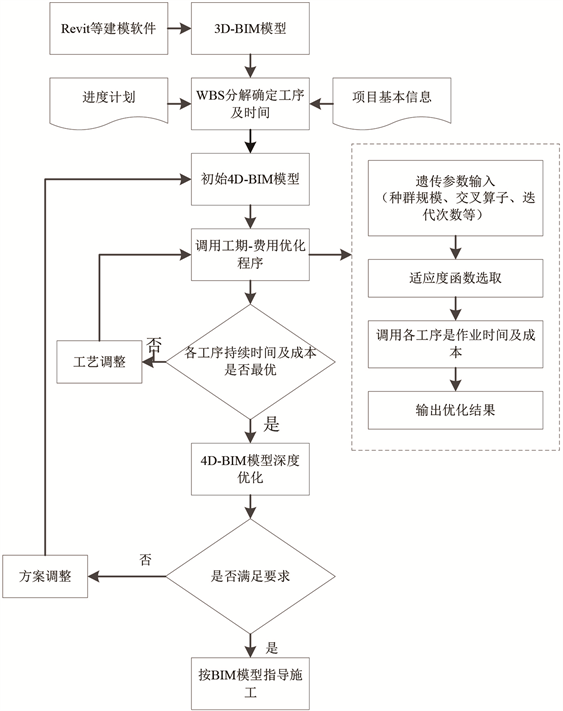Figure 1. Optimize the process

5. 实例验证

5.1. 工程概况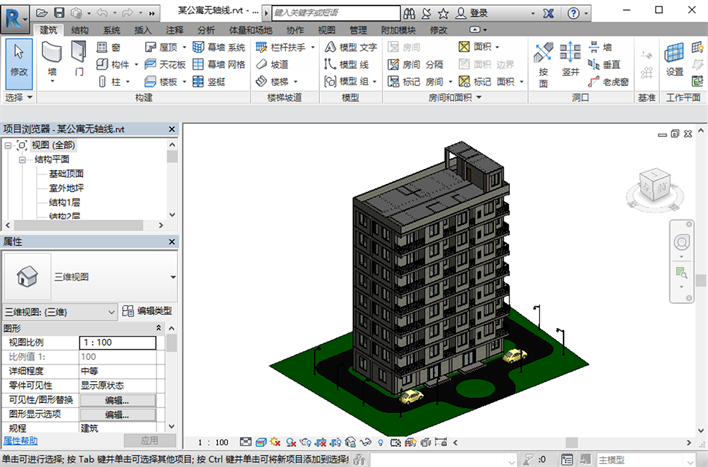Figure 2. 3D model of apartment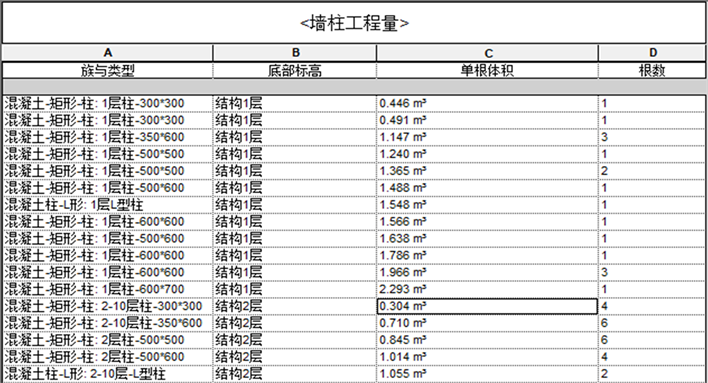Figure 3. Partial wall column scheduleTable 2. Apartment main structure construction process table

5.2. 算法应用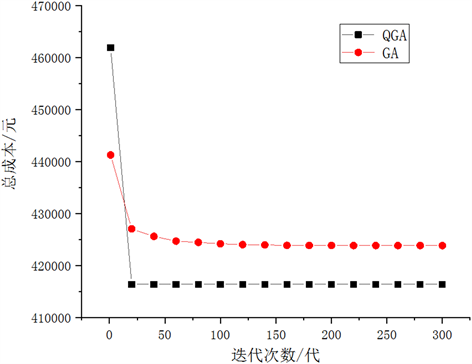Figure 4. Comparison of best fitness iteration curves

5.3. 仿真结果分析Table 3. Data comparison of simulation results

5.4. BIM技术模拟分析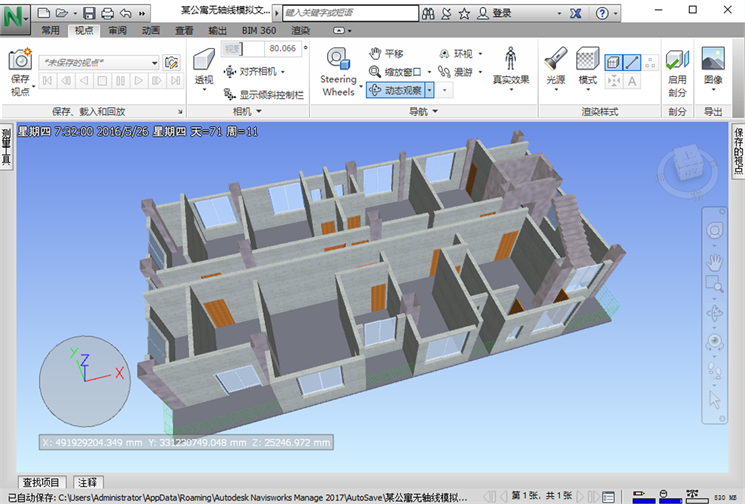Figure 5. Construction simulation

6. 结语

  杨凡. 基于遗传算法的工程项目工期——费用综合优化模型分析[D]: [硕士学位论文]. 郑州: 郑州大学, 2014.  胡长明, 熊焕军, 龙辉元, 等. 基于BIM的建筑施工项目进度–成本联合控制研究[J]. 西安建筑科技大学学报(自然科学版), 2014, 46(4): 474-478.  袁振民, 王要武. 基于BIM和网络计划技术的建设工程施工进度——成本研究[J]. 工程管理学报, 2015, 29(4): 95-100.  刘佳, 刘伊生, 施颖. 基于模糊集理论的施工项目工期–成本–质量权衡优化方法研究[J]. 北京交通大学学报(社会科学版), 2017, 16(3): 30-38.  Sun, J.D. and Wang, L. (2015) The Interaction between BIM’s Promotion and Interest Game under Information Asymmetry. Journal of Industrial and Management Opti-mization, 4, 1301-1319. https://doi.org/10.3934/jimo.2015.11.1301  刘文娟. 基于精益六西格玛管理的施工进度优化研究[D]: [硕士学位论文]. 西安: 西安建筑科技大学, 2015.  申建红, 刘丽丹, 孙小宁. 基于改进和声搜索算法的项目工期——成本优化[J]. 工程管理学报, 2017, 31(2): 121-125.  杨凯. 基于BIM技术工程项目4D进度优化研究[D]: [硕士学位论文]. 西安: 西安工业大学, 2017.  Lee, J.C., Lin, W.M., Liao, G.C., et al. (2011) Quantum Genetic Algorithm for Dynamic Economic Dispatch with Valve-Point Effects and Including Wind Power System. International Journal of Electrical Power & Energy Systems, 33, 189-197. https://doi.org/10.1016/j.ijepes.2010.08.014  Kong, H., Li, N. and Shen, Y. (2015) Adaptive Double Chain Quantum Genetic Algorithm for Constrained Optimization Problems. Chinese Journal of Aeronautics, 28, 214-228. https://doi.org/10.1016/j.cja.2014.12.010  程启明, 黄山, 张强, 等. 微电网三相负荷不平衡的量子遗传优化算法研究[J]. 电机与控制应用, 2017, 44(5): 56-62.  黄小庆, 杨夯, 陈颉, 等. 基于LCC和量子遗传算法的电动汽车充电站优化规划[J]. 电力系统自动化, 2015(17): 176-182.